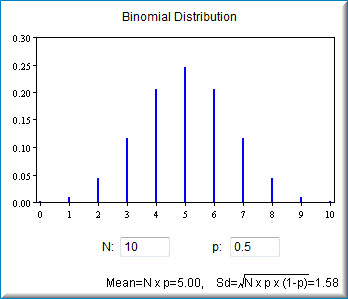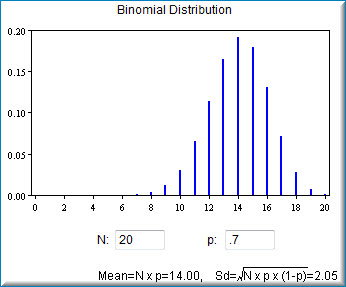# 5.8: Binomial Demonstration

•• Contributed by David Lane
• Associate Professor (Psychology, Statistics, and Management) at Rice University

Learning Objectives

• State the relationship between $$p$$ and skew.
• Compute the mean and standard deviation of a binomial distribution
• State how $$N$$ affects the shape of a binomial distribution

## Instructions

This demonstration allows you to explore the binomial distribution. Here you can specify the number of trials ($$N$$) and the proportion of successes ($$p$$). Note that the proportion of successes is sometimes referred to with the Greek letter $$p$$; here it will be referred to as $$p$$.

The starting values for $$N$$ and $$p$$ are $$10$$ and $$0.5$$. The graph therefore shows the probability of from $$0$$ to $$10$$ successes out of $$10$$ trials. The probability of $$0$$ successes is so small that it just barely shows up on the graph. The most likely number of successes is $$5$$, which has a probability of about $$0.25$$. Naturally, the sum of the probabilities is $$1.0$$.

The demonstration also shows the mean and the standard deviation of the binomial distribution.

## Illustrated Instructions

The screenshot below shows the binomial demonstration with its default data. You can adjust the number of trials ($$N$$) as well as the proportion of successes ($$p$$).Figure $$\PageIndex{1}$$: Binomial demonstration with default data

The example below shows a distribution of $$20$$ trials with a probability of success of $$0.7$$. Notice that the mean and standard deviation for the distribution are also shown.Figure $$\PageIndex{1}$$: Binomial distribution of 20 trials

## Contributor

• Online Statistics Education: A Multimedia Course of Study (http://onlinestatbook.com/). Project Leader: David M. Lane, Rice University.